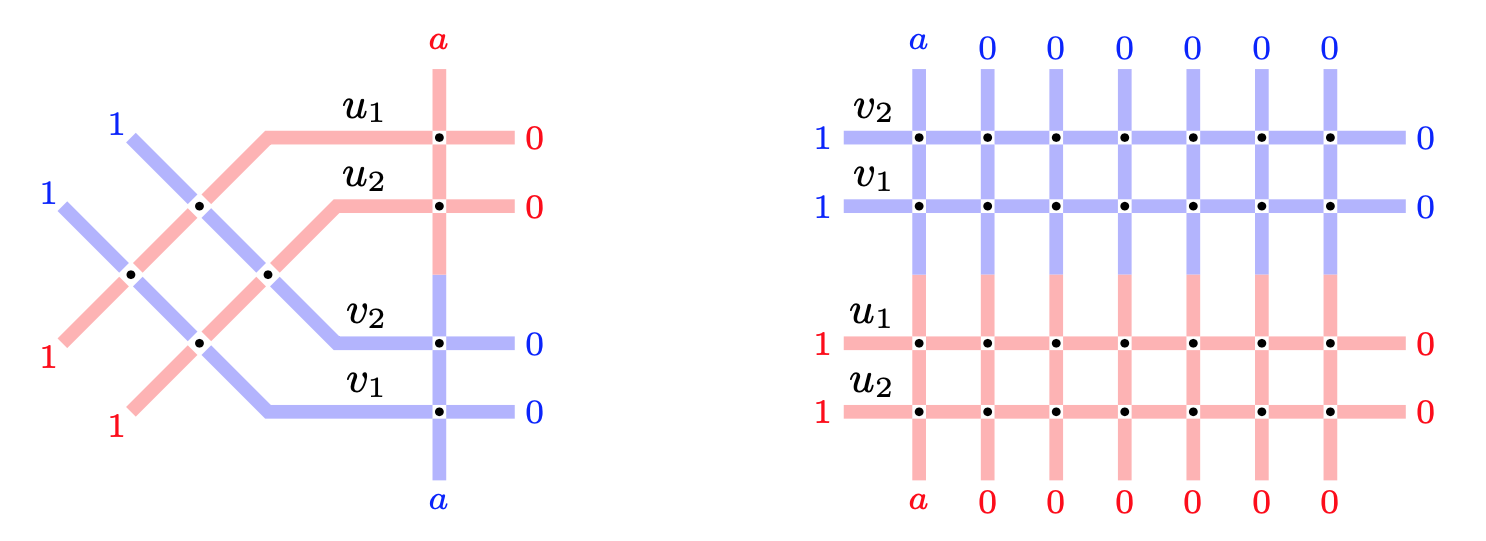### Refined Cauchy identity for spin Hall-Littlewood symmetric rational functions

[2020/07/20]

Fully inhomogeneous spin Hall-Littlewood symmetric rational functions $\mathsf{F}_\lambda$ arise in the context of $\mathfrak{sl}(2)$ higher spin six vertex models, and are multiparameter deformations of the classical Hall-Littlewood symmetric polynomials. We obtain a refined Cauchy identity expressing a weighted sum of the product of two $\mathsf{F}_\lambda$’s as a determinant. The determinant is of Izergin-Korepin type: it is the partition function of the six vertex model with suitably decorated domain wall boundary conditions. The proof of equality of two partition functions is based on the Yang-Baxter equation.

We rewrite our Izergin-Korepin type determinant in a different form which includes one of the sets of variables in a completely symmetric way. This determinantal identity might be of independent interest, and also allows to directly link the spin Hall-Littlewood rational functions with (the Hall-Littlewood particular case of) the interpolation Macdonald polynomials. In a different direction, a Schur expansion of our Izergin-Korepin type determinant yields a deformation of Schur symmetric polynomials.

In the spin-$\frac12$ specialization, our refined Cauchy identity leads to a summation identity for eigenfunctions of the ASEP (Asymmetric Simple Exclusion Process), a celebrated stochastic interacting particle system in the Kardar-Parisi-Zhang universality class. This produces explicit integral formulas for certain multitime probabilities in ASEP.

### Online conference on Statistical Mechanics, Integrable Systems and Probability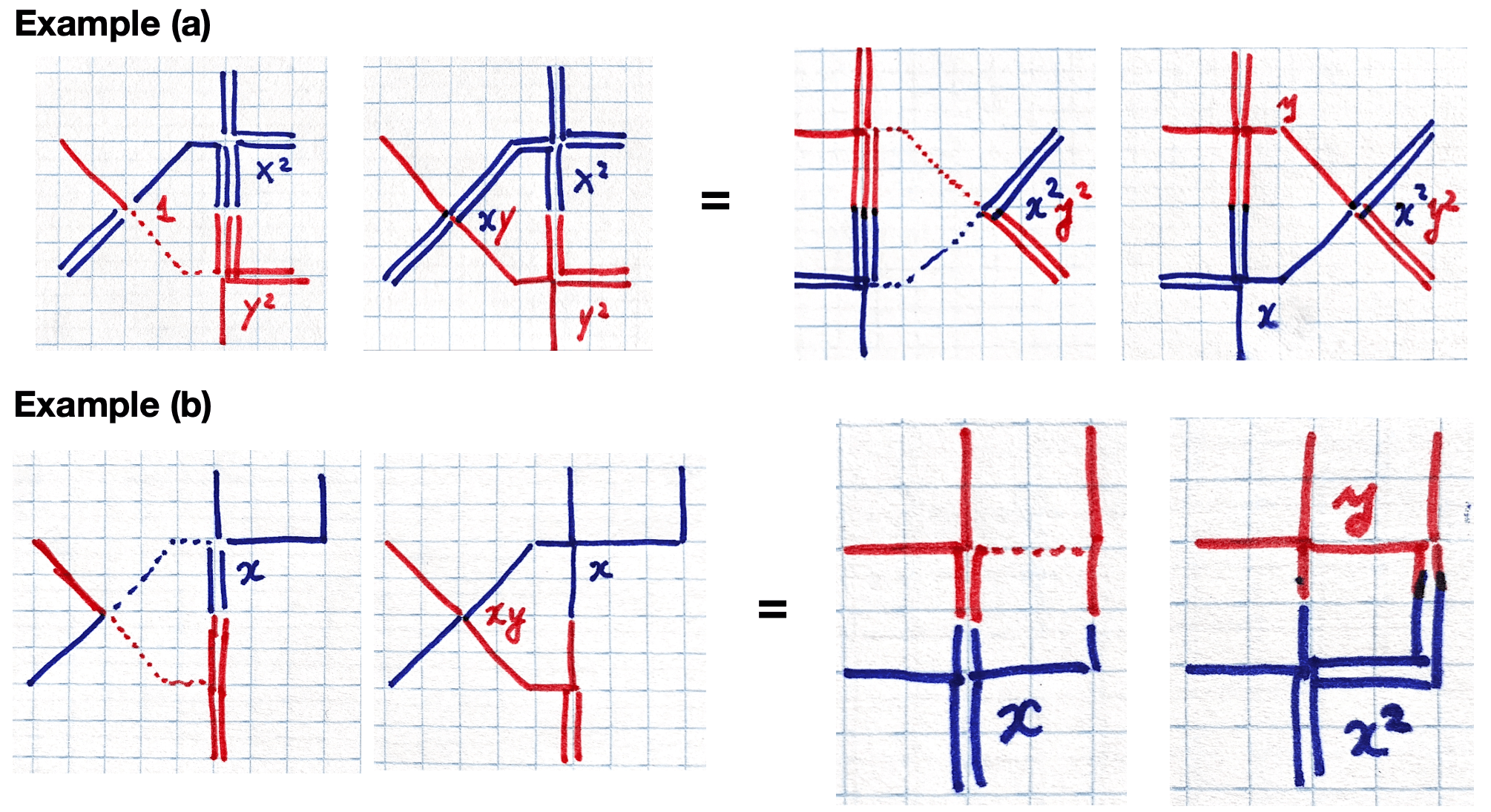### Spin deformation of q-Whittaker polynomials and Whittaker functions

Spin $q$-Whittaker symmetric polynomials labeled by partitions $\lambda$ were recently introduced by Borodin and Wheeler (arXiv:1701.06292) in the context of integrable $\mathfrak{sl}_2$ vertex models. They are a one-parameter deformation of the $t=0$ Macdonald polynomials. We present a new, more convenient modification of spin $q$-Whittaker polynomials and find two Macdonald type $q$-difference operators acting diagonally in these polynomials with eigenvalues, respectively, $q^{-\lambda_1}$ and $q^{\lambda_N}$ (where $\lambda$ is the polynomial’s label). We study probability measures on interlacing arrays based on spin $q$-Whittaker polynomials, and match their observables with known stochastic particle systems such as the $q$-Hahn TASEP.

In a scaling limit as $q\nearrow 1$, spin $q$-Whittaker polynomials turn into a new one-parameter deformation of the $\mathfrak{gl}_n$ Whittaker functions. The rescaled Pieri type rule gives rise to a one-parameter deformation of the quantum Toda Hamiltonian. The deformed Hamiltonian acts diagonally on our new spin Whittaker functions. On the stochastic side, as $q\nearrow 1$ we discover a multilevel extension of the beta polymer model of Barraquand and Corwin (arXiv:1503.04117), and relate it to spin Whittaker functions.

Based on a joint work with Matteo Mucciconi and earlier works with Alexey Bufetov and both of them.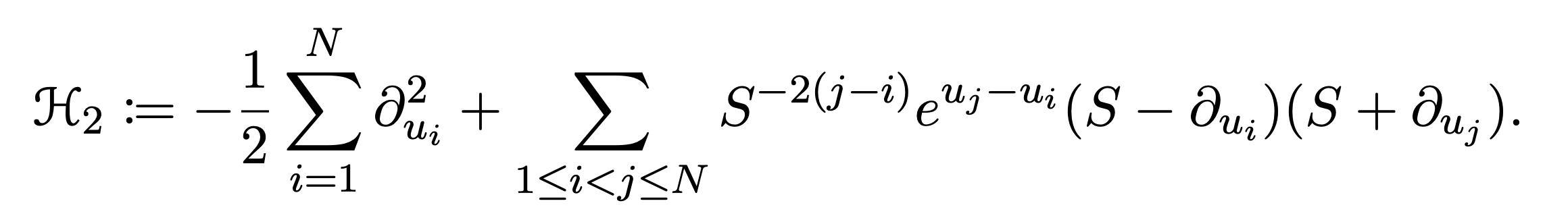### Spin q-Whittaker polynomials and deformed quantum Toda

[2020/03/31]

Spin $q$-Whittaker symmetric polynomials labeled by partitions $\lambda$ were recently introduced by Borodin and Wheeler (arXiv:1701.06292) in the context of integrable $\mathfrak{sl}_2$ vertex models. They are a one-parameter deformation of the $t=0$ Macdonald polynomials. We present a new, more convenient modification of spin $q$-Whittaker polynomials and find two Macdonald type $q$-difference operators acting diagonally in these polynomials with eigenvalues, respectively, $q^{-\lambda_1}$ and $q^{\lambda_N}$ (where $\lambda$ is the polynomial’s label). We study probability measures on interlacing arrays based on spin $q$-Whittaker polynomials, and match their observables with known stochastic particle systems such as the $q$-Hahn TASEP.

In a scaling limit as $q\nearrow 1$, spin $q$-Whittaker polynomials turn into a new one-parameter deformation of the $\mathfrak{gl}_n$ Whittaker functions. The rescaled Pieri type rule gives rise to a one-parameter deformation of the quantum Toda Hamiltonian. The deformed Hamiltonian acts diagonally on our new spin Whittaker functions. On the stochastic side, as $q\nearrow 1$ we discover a multilevel extension of the beta polymer model of Barraquand and Corwin (arXiv:1503.04117), and relate it to spin Whittaker functions.

### 2021 travel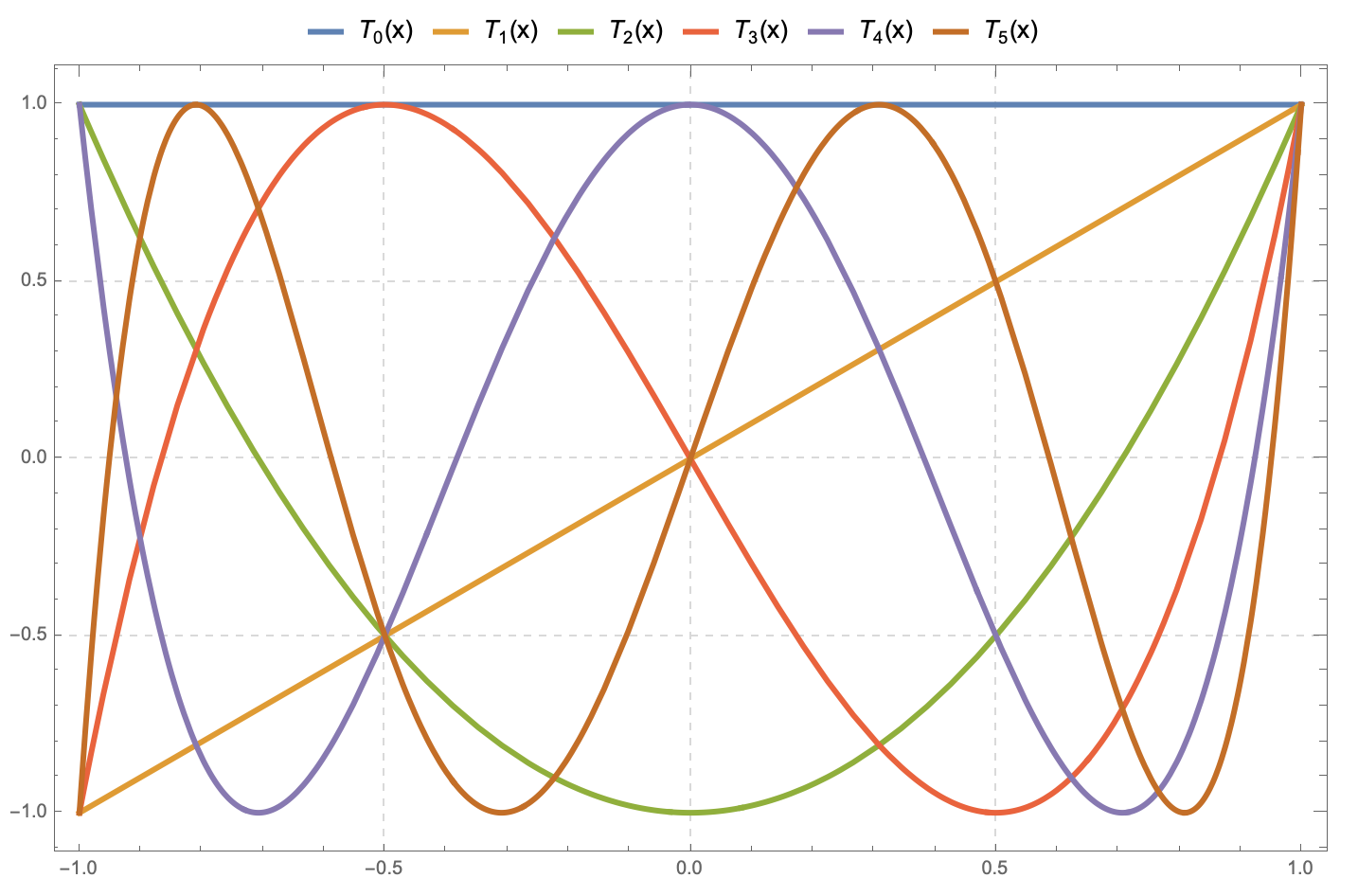### MATH 7310 • Real Analysis and Linear Spaces I

[Spring 2020 semester]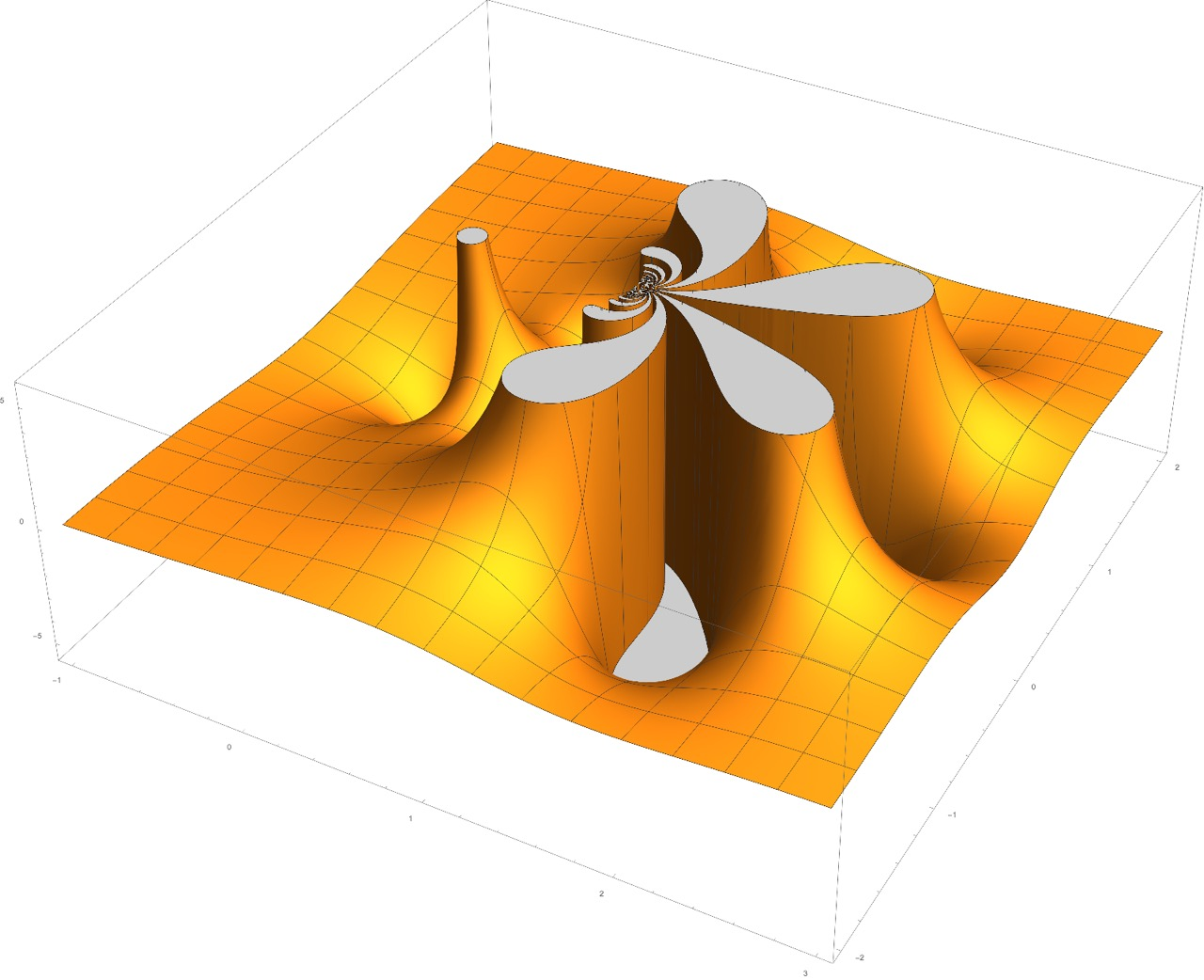### MATH 3340 • Complex Variables with Applications

[Spring 2020 semester]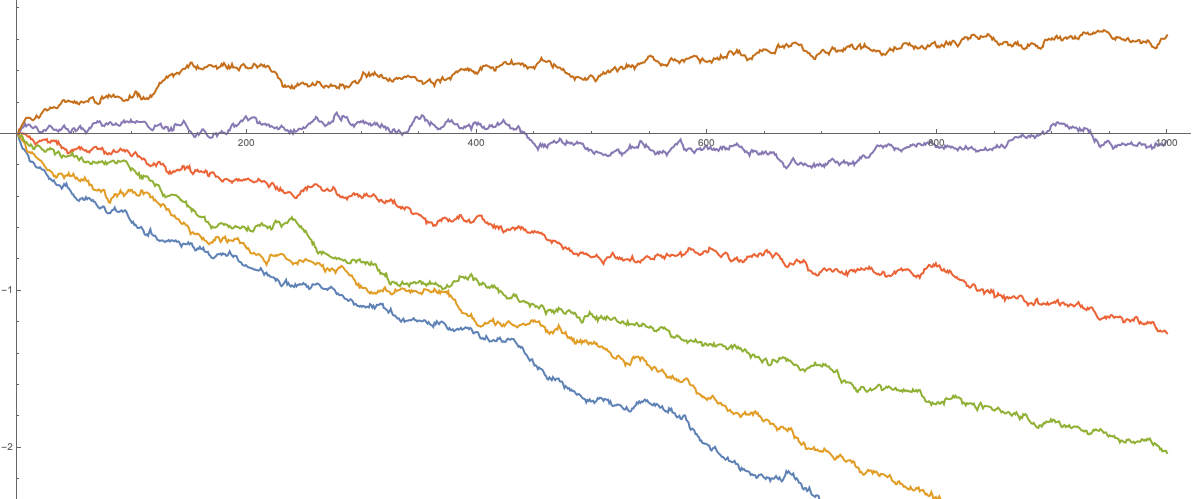### Parameter symmetry in perturbed GUE corners process and reflected drifted Brownian motions

[2019/12/17]

The perturbed GUE corners ensemble is the joint distribution of eigenvalues of all principal submatrices of a matrix $G+\mathrm{diag}(\mathbf{a})$, where $G$ is the random matrix from the Gaussian Unitary Ensemble (GUE), and $\mathrm{diag}(\mathbf{a})$ is a fixed diagonal matrix. We introduce Markov transitions based on exponential jumps of eigenvalues, and show that their successive application is equivalent in distribution to a deterministic shift of the matrix. This result also leads to a new distributional symmetry for a family of reflected Brownian motions with drifts coming from an arithmetic progression.

The construction we present may be viewed as a random matrix analogue of the recent results of the first author and Axel Saenz.